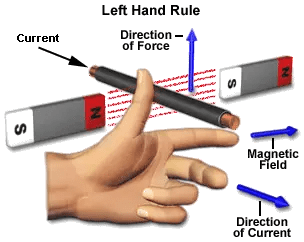While permanent magnets produce a good and sometimes very strong static magnetic field, in some applications the strength of this magnetic field is still too weak or we need to be able to control the amount of magnetic flux that is present. So in order to produce a much stronger and more controllable magnetic field we need to use electricity.

By using coils of wire wrapped or wound around a soft magnetic material such as an iron core we can produce very strong electromagnets for use in many different types of electrical applications. This use of coils of wire produces a relationship between electricity and magnetism that gives us another form of magnetism called Electromagnetism.

Electromagnetism is produced when an electrical current flows through a simple conductor such as a length of wire or cable, and as current passes along the whole of the conductor then a magnetic field is created along the whole of the conductor. The small magnetic field created around the conductor has a definite direction with both the “North” and “South” poles produced being determined by the direction of the electrical current flowing through the conductor.

Therefore, it is necessary to establish a relationship between current flowing through the conductor and the resultant magnetic field produced around it by this flow of current allowing us to define the relationship that exists between Electricity and Magnetism in the form of Electromagnetism.

We have established that when an electrical current flows through a conductor a circular electromagnetic field is produced around it with the magnetic lines of flux forming complete loops that do not cross around the whole length of the conductor. The direction of rotation of this magnetic field is governed by the direction of the current flowing through the conductor with the corresponding magnetic field produced being stronger near to the center of the current carrying conductor. This is because the path length of the loops being greater the further away from the conductor resulting in weaker flux lines as shown below.

Any change in the magnetic field of a coil of wire will cause an emf to be induced in the coil. This emf induced is called induced emf and if the conductor circuit is closed, the current will also circulate through the circuit and this current is called induced current. Method to change magnetic field:

1. By moving a magnet towards or away from the coil
2. By moving the coil into or out of the magnetic field.
3. By changing the area of a coil placed in the magnetic field
4. By rotating the coil relative to the magnet.It is found that whenever an current carrying conductor is placed inside a magnetic field, a force acts on the conductor, in a direction perpendicular to both the directions of the current and the magnetic field. In the figure it is shown that, a portion of a conductor of length L placed vertically in a uniform horizontal magnetic field strength H, produced by two magnetic poles N and S. If i is the current flowing through this conductor, the magnitude of the force acts on the conductor is, F = BiLHold out your left hand with forefinger, second finger and thumb at right angle to one another. If the fore finger represents the direction of the field and the second finger that of the current, then thumb gives the direction of the force. While, current flows through a conductor, one magnetic field is induced around it. This can be imagined by considering numbers of closed magnetic lines of force around the conductor. The direction of magnetic lines of force can be determined by Maxwell’s corkscrew rule or right-hand grip rule. As per these rules, the direction of the magnetic lines of force (or flux lines) is clockwise if the current is flowing away from the viewer, that is if the direction of current through the conductor is inward from the reference plane as shown in the figure.As per Faraday’s law of electromagnetic induction, whenever a conductor moves inside a magnetic field, there will be an induced currentin it. If this conductor gets forcefully moved inside the magnetic field, there will be a relation between the direction of applied force, magnetic field and the current. This relation among these three directions is determined by Fleming Right Hand Rule This rule states “Hold out the right hand with the first finger, second finger and thumb at right angle to each other. If forefinger represents the direction of the line of force, the thumb points in the direction of motion or applied force, then second finger points in the direction of the induced current.